## Power of a Matrix

A repeat multiplication of a square matrix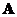with itself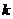times produces matrix power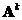, thus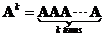When the poweris large, computation of matrix multiplication takes a long time and a more efficient method is to transform matrixinto a similar diagonal matrix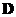using similarity transformation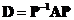. Matrix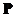(is called modal matrix) is formed by horizontal concatenation of the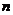linearly independent eigenvectors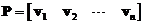. Matrixcan be obtained back from diagonal matrix using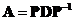. The computation of matrix power is simplified by taking the power to the scalar diagonal elements. Then multiply the diagonal power with the modal matrix and its inverse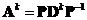.

When the poweris not integer the computation of matrix power is possible only through similarity transformation into diagonal matrix . The diagonalization can take place only if the modal matrixhas an inverse matrix .

Example:
From matrix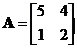we can obtain diagonal matrix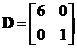. Through modal matrix (augmented eigenvectors)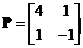. The inverse modal matrix is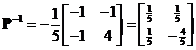.

Then, matrix power can be computed through repeated matrix multiplication 4 times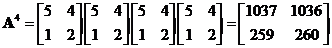. The same result can be obtained using diagonal matrix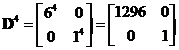. Then we multiply with modal matrix and its inverse, require only three matrix multiplications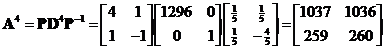.
Clearly this procedure is only more efficient than matrix multiplication when the poweris large.

However, we have something else that matrix multiplication cannot perform: when matrix poweris not integer.

Example:
Using the matrices in previous example, find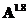In this example, matrix multiplication cannot solve our problem.
Solution: We can take the exponent to the diagonal elements of the diagonal matrix as in scalar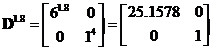. We multiply back to obtain the matrix power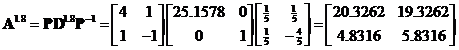.